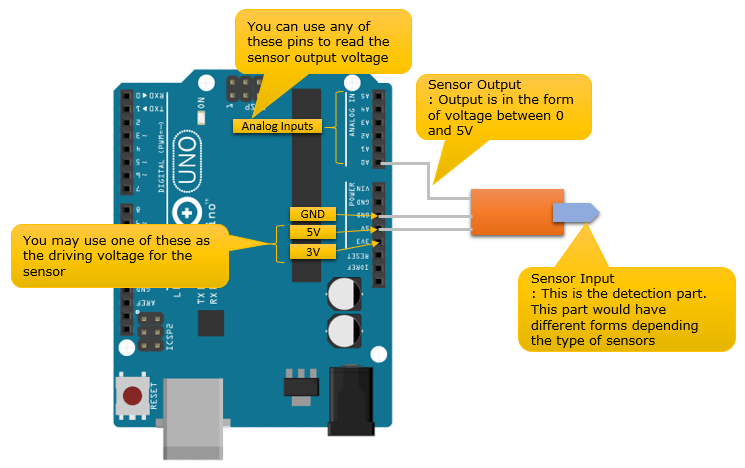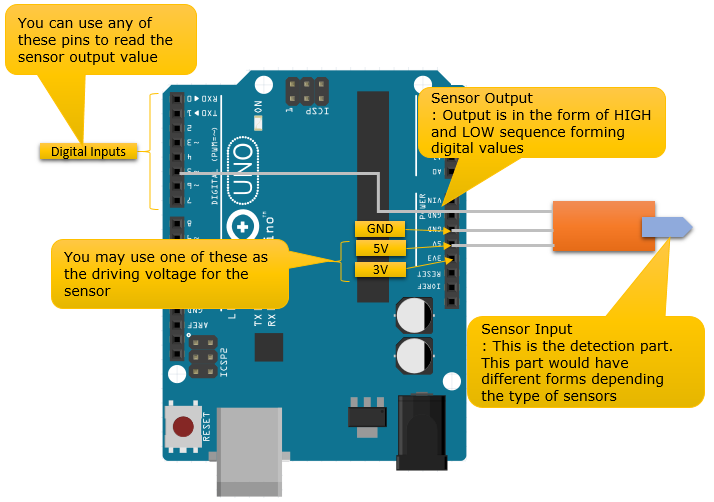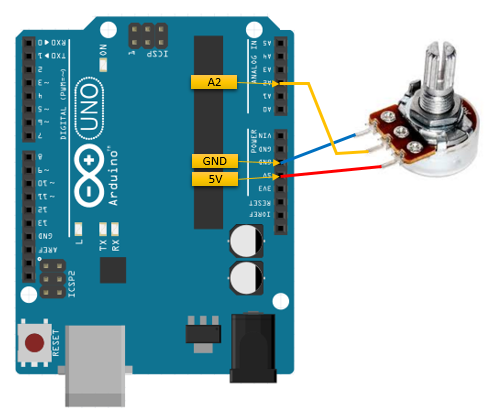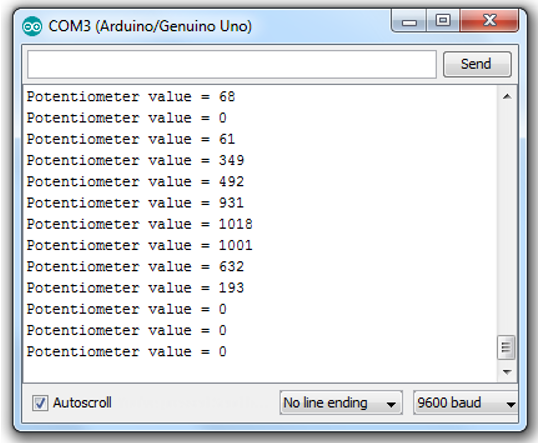Embedded System - Arduino Sensor Basic   In this page, I will explain about how you operate various sensors with Arduino board. There are whole a lot of different sensors you can play with Arduino. Even though all those sensors would have different functionalities, the basic operation and circuitary is almost same as shown below regardless of what kind of sensor they are. Actually, you need to build a circuit around the sensors to make it possible to operate using the following circuit. However, if you purchase any Arduino compatitble sensor module, the module itself already built all the necessary cuircuits on the module to make following connection possible. So, all you need to do is just to do a couple of wiring and write a couple of lines of code.   Roughly there are two types of sensor. One is Analog Sensor that measure some thing and generate output (sensor value) in the form of analog signal (analog voltage) and the other one is Digital Sensor that enerate output (sensor value) in the form of digital signal. Personally, I would recommend you to use Analog sensors if you have choice because it would be easier with programming.  If you use digital sensors, you need to do a little bit complicated programming to process the sequence of digital pulses (serial data) into a value. (You don't have to worry too much about this since most of the digital sensor module provide you their own C API (function) that does all these processing for you. In terms of Arduino programming, it would not be much different from Analog Sensor program. However, if you are a type of person who wants to understand every details whatever it is, you would need to invest pretty much of time and effort to understand the C API provided by the sensor maker or any open-source project)   Following shows the typical circuit for Analog sensors.Following shows the typical circuit for Digital sensors.Before you buy a sensor module   I think most of Arduino compatible sensor module are already designed in such a way that can electrically fit to the cuircuit shown above, but I would suggest you to check some basic things as follows before you buy a sensor module.   How much voltage is required to operate (drive) the sensor module ? : If it requires 5V or 3V, it would be good since Arduino has 5V and 3V output. (If you really want to use a sensor module which requires much higher or lower voltage to drive, you need to build a small external circuit that can convert the 3V or 5V into whatever voltage that the module requires). How much current is required to operate (drive) the sensor module ? : As you see in 'First Thing You need to know of Arduino Pins', 3V output can supply the max current of 50 mA and 5V output can supply the max current of several hundreds of mV (it can supply around 500 mA max in most case). So it would be better to purchase the sensor module that can operate within this range of current. (If it is Analog Sensor), What is the range of the output voltage of the sensor module ? : Arduno Analog input can read the voltage within the range of 0~5 V. So, it would be better to buy the module with similar range of output voltage. However you also need to be careful not to purchase the module with too small output. Arduino analog input pin read 0~5V value with 0~1023 values. It means the mininum voltage that a pin can read as a meaningful value is about 5 mV (= 5000mV/1024).       Potentiometer   In this section, I will show you how to use (operate) a potentiometer with Arduino. I picked this one as the first example since it would be the simplest and probably the cheapest one you can get. You can connect a Potentiometer as shown below. I used the A2 pin in this example, but you can use any Analog pins as you want. After I read the potentiometer value, I will send the data to the PC that is connected to Arduino via serial port just for simplicity. So you'd better understand how to use Serial port in Arduino before you go through this example (Refer to Serial Comm page on how Serial communication works in Arduino )Following is the Arduino Code for this example. As you see, it is very simple... just read the value from A2 pin and write the value through the serial port.   int potentiometerValue = 0; // This variable is to store the sensor value that was read through the analog pin char potOut; // This variable is to store the string containing the sensor value   void setup() {   // Initialize the serial port with the baud rate of 9600   Serial.begin(9600); }   void loop() {         // Read the analog input value from A2 pin and store the result to potentiometerValue         potentiometerValue = analogRead(A2);           // create a string that contains the sensor value and some additional text. As you see here,         // you can use the standard C language funtion in Arduino code.         // At first I tried "String potOut = "Potentiometer value =" + analogRead(A2);", but somehow this         // sent a strange looking string. So I used the native C function.         sprintf(potOut,"Potentiometer value = %d",potentiometerValue);           // sent the string via Serial port         Serial.println(potOut);           // sleep 1 sec         delay(1000); }   Run the code and rotate the knob of the potentiometer as the program is running. You would get the result something as follows. (The exact value may differ from what you get, but you see some changing values as you rotate the knob)Temperature / Humidity Sensor   Since this type of sensor would be pretty complicated to understand the details and the page would be pretty long, I posted it in a separate page : titled as DHT.     Light Sensor   I got a simple light sensor from OSEPP which detect the intensity of light around it and output the result as in the form of analog signal. I am posting the tutorial in a separate page : titled as Light Sensor